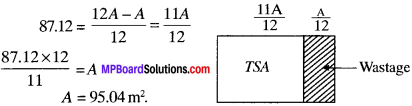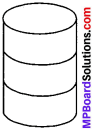# MP Board Class 9th Maths Solutions Chapter 13 Surface Areas and Volumes Ex 13.2

## MP Board Class 9th Maths Solutions Chapter 13 Surface Areas and Volumes Ex 13.2

Assume π = $$\frac{22}{7}$$ unless stated otherwise.

Question 1.
The curved surface area of a right circular cylinder of height 14 cm is 88 cm2. Find the diameter of the base of the cylinder.
Solution:
h = 14 cm
CSA of cylinder = 88 cm2
CSA of cylinder = 2 πrh
88 = 2x $$\frac{22}{7}$$ xr x 14
$$\frac{88}{4}$$ = 2r
r = $$\frac{2}{2}$$ = 1
Diameter = 2 r
= 1 x 2 = 2 cm.Question 2.
It is required to make a closed cylindrical tank of height 1 m and base diameter 140cm from a metal sheet. How many square metres of the sheet are required for the same?
h = 1 m
d = 140 cm
r = 7o cm = 0.7 m
Area of metal required = TSA of cylinderical tank
= 2 πr (r + h)
= 2 x $$\frac{22}{7}$$ x 0.7 (0.7 + 1)
= 4.4 x 1.7 = 7.4m2

Question 3.
A metal pipe is 77 cm long. The inner diameter of a cross section is 4 cm. the outer diameter being 4.4 cm. (see Fig.). Find its

(i) inner curved surface area.
(ii) outer curved surface area.
(iii) total surface area.(iii) TSA = ICSA + OCSA + 2 x area of ring
= 968 + 1064.8 + 2 x 2.64
= 2032.8 + 5.28
= 20.38.08 cm2.

Question 4.
The diameter of a roller is 84 cm and its length is 120 cm. It takes 500 complete revolutions to move once over to level a playground. Find the area of the playground in m2.
Solution:
d = 84cm
∴ r = 42 cm
h = 120 cm
No. of revolution = 500
CSA of the roller = 2πrh
= 2 x $$\frac{22}{7}$$ x 42 x 120
= 44 x 720
= 31680 cm2
Area of the playground = 31680 x 500
= 15840000 cm2
Area in m2 $$\frac{ 15840000}{100 x 100}$$
= 1584 m2.Question 5.
A cylindrical pillar is 50 cm in diameter and 3.5 m in height Find the cost of painting the curved surface of the pillar at the rate of ₹ 12.S0 per m2.
Solution:
d = 50cm
r = 25 cm2
h = 3.5 m = 350 cm
CSA of pillar = 2πrh
= 2x $$\frac{22}{7}$$ x 25 x 350
= 44 x 1250
= 55000 cm2
CSA in m2 = $$\frac{55000}{100×100}$$
Cost of painting the cylindrical pillar = ₹ 12.50 x 5.5
= ₹ 68.75

Question 6.
Curved surface area of a right circular cylinder is 4.4 m2. If the radius of the base of the cylinder is 0.7 m, find its height.
Solution:
CSA = 4.4m2.
r = 0.7 m
CSA of cylinder =2πrh
4.4 = 2x $$\frac{22}{7}$$ x 0.7 x h
4.4 = 44 x 0.1 x h
4.4 = 4.4 x h
h = $$\frac{44}{4.4}$$ = 1m

Question 7.
The inner diameter of a circular well is 3.5 m. It is 10 m deep. Find

1. its inner curved surface area
2. the cost of plastering this curved surface at the rate of ₹ 40 per m2.

Solution:
d = 3.5m
r = 1.75 m
h = 10 m

1. ICSA of the well = 2πrh
= 2 x $$\frac{22}{7}$$ x 1.75 x 10
= 110 m2

2. Cost of plastering = ₹ 40 x 110
= ₹ 4400.Question 8.
In a hot water heating system, there is a cylindrical pipe of length 28 m and diameter 5 cm. Find the total radiating surface in the system.
Solution:
h = 28m
d = 5cm
r = 2.5cm = 0.025m
Total radiating surface = CSA of pipe = 2πrh
= 2 x $$\frac{22}{7}$$ x 0.025 x 28
= 4.4 m2.

9. Find

1. The lateral or curved surface area of a closed cylindrical petrol storage tank that is 4.2 m in diameter and 4.5 m high.

2. How much steel was actually used, if $$\frac{1}{2}$$ of the steel actually used was wasted in making the tank.

Solution:
1. d = 4.2 m
r = 2.1m
h = 4.5 m
CSA of cylinderical tank = 2πrh
= 2x $$\frac{22}{7}$$ x 2.1 x 4.5
= 59.4 m2.
TSA = 2πrr(r + h)
= 2 x $$\frac{22}{7}$$ x 2.1 (2.1 + 4.5)
= 2 x $$\frac{22}{7}$$ x 2.1 x 6.6
= 44 x 1.98
= 87.12 m2

2. Let A be the area of sheet actually used
TSA = A – $$\frac{1}{12}$$A = 95.04m2.

Question 10.
In Fig. you see the frame of a lampshade. It is to be covered with a decorative cloth. The frame has a base diameter of 20 cm and height of 30 cm. Amargin of 2.5 cm is to be given for folding it over the top and bottom of the frame. Find how much cloth is required for covering the lampshade.
Solution:d = 20 cm
r = 10 cm
h = 30 + 2.5 + 2.5 cm = 35 cm
Area of cloth required = CSA of cyliner of height 35 cm
= 2πrh
= 2 x $$\frac{22}{7}$$ x 10 x 35
= 44 x 50 = 2200 cm2.Question 11.
The students of a Vidyalaya were asked to participate in a competi-tion for making and decorating penholders in the shape of a cylinder with a base, using cardboard. Each penholder was to be of radius 3 cm and height 10.5 cm. The Vidyalaya was to supply the competitors with cardboard. If there were 35 competitors, how much cardboard was required to be bought for the competition?
Solution:
r = 3 cm
h = 10.5 cm
Cardboard required for one penholder = CSA of penholder + Area of base
= 2πrh + πr2